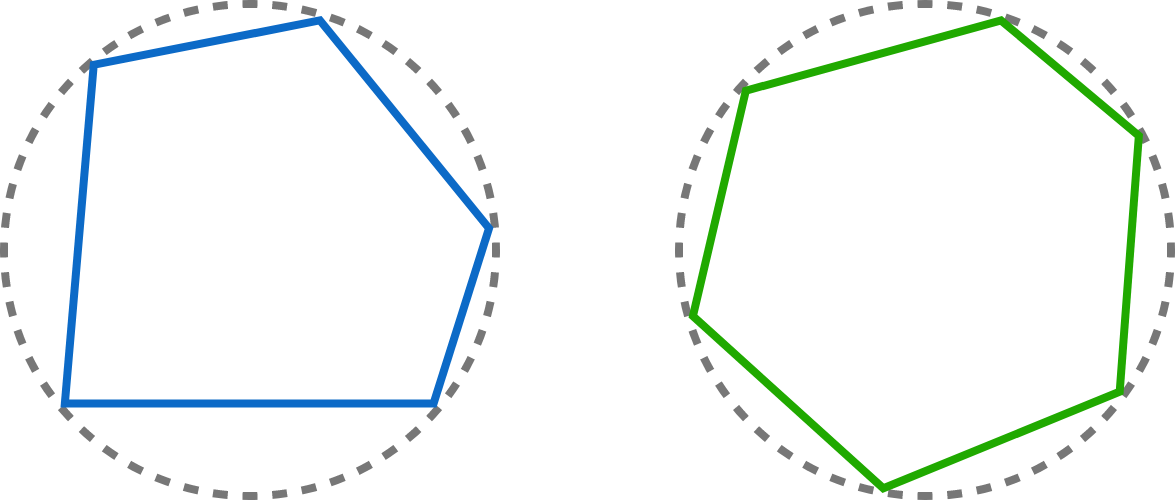# Equal Angles = Equal Sides?

Geometry Level 3

True or False?

1. A cyclic $\color{#3D99F6}\text{pentagon}$ has equal angles if and only if it has equal sides.
2. A cyclic $\color{#20A900} \text{hexagon}$ has equal angles if and only if it has equal sides.


Note: A cyclic polygon is a polygon that can be inscribed in a circle. A cyclic pentagon and a cyclic hexagon are shown below.×